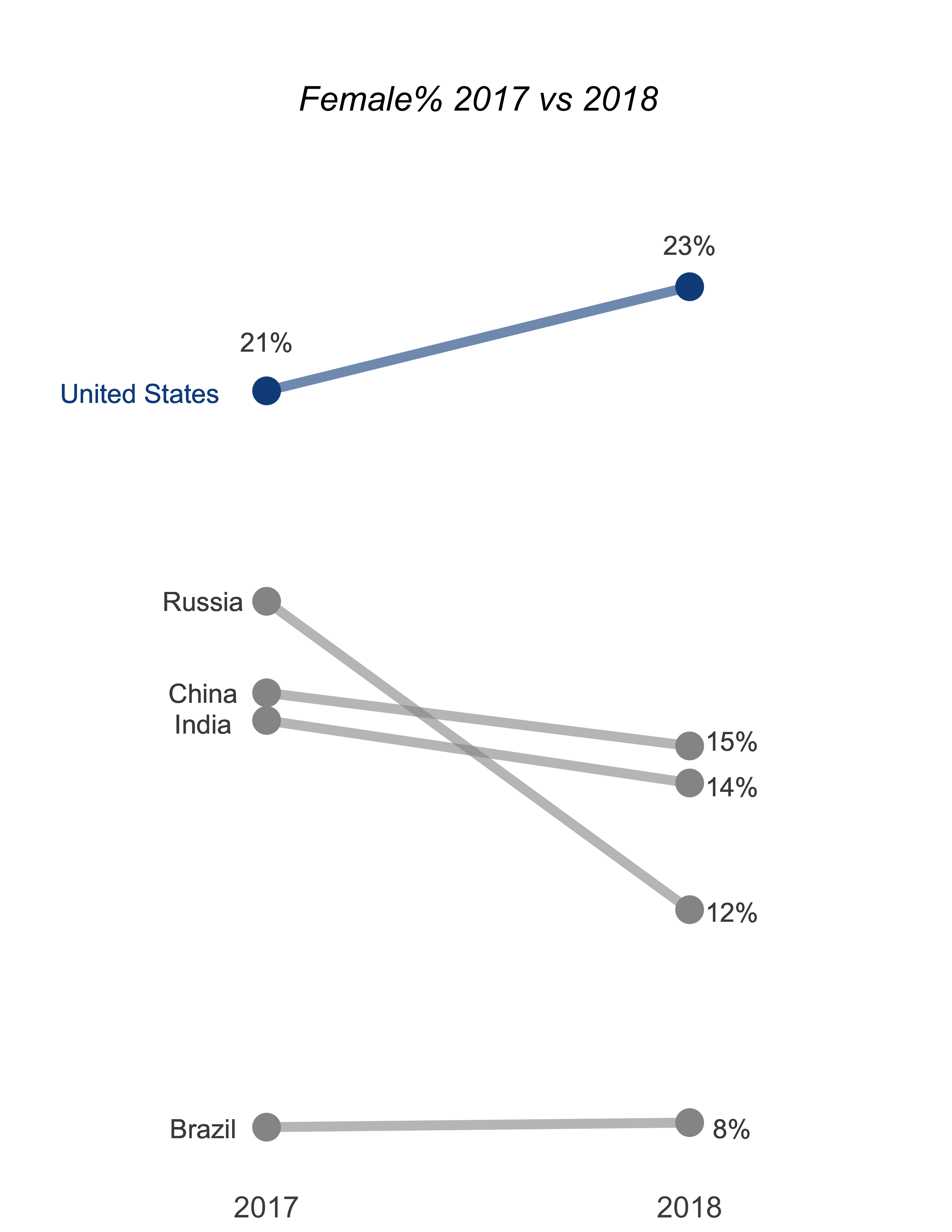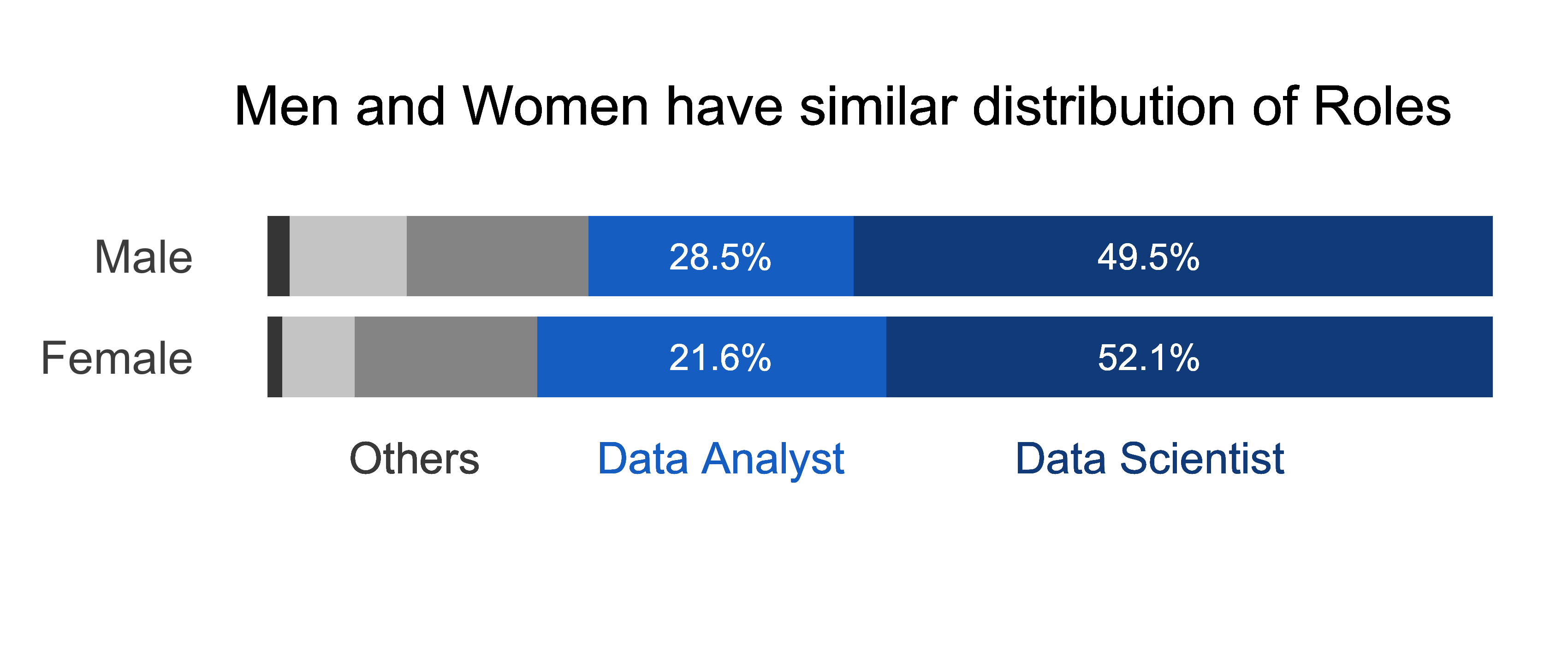## Gender Divide in Data Science

``````    Aakanksha Nallabothula Surya
Shreejaya Bharathan``````
In :
``````%reload_ext rpy2.ipython
%R library(ggplot2)
%R library(scales)
import numpy as np
import pandas as pd``````
In :
``````kaggle_2018_data = pd.read_csv('./kaggle-survey-2018/multipleChoiceResponses.csv')
kaggle_2018_data.head()
``````
```/Users/aakanksha/miniconda3/lib/python3.7/site-packages/IPython/core/interactiveshell.py:3058: DtypeWarning: Columns (0,2,8,10,21,23,24,25,26,27,28,44,56,64,83,85,87,107,109,123,125,150,157,172,174,194,210,218,219,223,246,249,262,264,276,277,278,279,280,281,282,283,284,285,286,287,288,289,290,304,306,325,326,329,341,368,371,384,385,389,390,391,393,394) have mixed types. Specify dtype option on import or set low_memory=False. interactivity=interactivity, compiler=compiler, result=result) ```
Out:
In :
``````kaggle_2017_data = pd.read_csv('multipleChoiceResponses_2017.csv', encoding = "ISO-8859-1")
kaggle_2017_data.head()
``````
```/Users/aakanksha/miniconda3/lib/python3.7/site-packages/IPython/core/interactiveshell.py:3058: DtypeWarning: Columns (31,83,86,87,98,99,109,116,123,124,127,129,130,164) have mixed types. Specify dtype option on import or set low_memory=False. interactivity=interactivity, compiler=compiler, result=result) ```
Out:

### Data Cleaning

``````Only select Male and Female Data

Remove all responses which took less than 5 minutes to complete (Long survey, genuine response would take
longer than 5 minutes)``````
In :
``````data_2018 = kaggle_2018_data.loc[(kaggle_2018_data.Q1 == 'Female') | (kaggle_2018_data.Q1 == 'Male')]
data_2018['Time from Start to Finish (seconds)'] = pd.to_numeric(data_2018['Time from Start to Finish (seconds)'])
data_2018 = data_2018.loc[data_2018['Time from Start to Finish (seconds)']>500]``````
```/Users/aakanksha/miniconda3/lib/python3.7/site-packages/ipykernel_launcher.py:2: SettingWithCopyWarning: A value is trying to be set on a copy of a slice from a DataFrame. Try using .loc[row_indexer,col_indexer] = value instead See the caveats in the documentation: http://pandas.pydata.org/pandas-docs/stable/user_guide/indexing.html#returning-a-view-versus-a-copy ```
In :
``data_2017 = kaggle_2017_data.loc[(kaggle_2017_data.GenderSelect == 'Female') | (kaggle_2017_data.GenderSelect == 'Male')]``

### Graph 1 - % Female vs Male Respondents

Waffle Graph

In :
``````# finding the percentage of female respondents
graph1 = data_2018[['Q1','Q4']]
graph1 = round(100*graph1.groupby('Q1').count()/graph1.shape).reset_index()
graph1
``````
Out:
In :
``%R -i graph1``
In :
``%R library(waffle)``
Out:
``````array(['waffle', 'scales', 'ggplot2', 'tools', 'stats', 'graphics',
'grDevices', 'utils', 'datasets', 'methods', 'base'], dtype='<U9')``````
In :
``````%%R -w 8.5 -h 11 --units in -r 400

ggplot(data = graph1, aes(fill = Q1, values = Q4)) +
geom_waffle(n_rows = 10, size = 0.5, colour = "#ffffff",  flip = FALSE, use_glyph = c("female", "male")) +
scale_fill_manual(values = c("deepskyblue4","grey56")) +
coord_equal() +
theme_minimal() +
theme_enhance_waffle() +
labs(title = "% Female Respondents ",
subtitle = "Kaggle ML & DS Survey 2018")+
theme(plot.title = element_text(size = 22,hjust = 0.5),
plot.subtitle = element_text(size = 18,hjust = 0.5),
legend.title = element_blank())
#ggsave('./percent_women.pdf', units = 'in', width = 8.5, height = 11)``````
```R[write to console]: Error in geom_waffle(n_rows = 10, size = 0.5, colour = "#ffffff", flip = FALSE, : could not find function "geom_waffle" Calls: <Anonymous> -> <Anonymous> -> withVisible ```
``` Error in geom_waffle(n_rows = 10, size = 0.5, colour = "#ffffff", flip = FALSE, : could not find function "geom_waffle" Calls: <Anonymous> -> <Anonymous> -> withVisible ```

### Graph 2 - Percent women per country 2017 vs 2018

Slope Graph

In :
``````countries_2017 = data_2017[['GenderSelect', 'Country']]
countries_2017['count'] = 1``````
```/Users/aakanksha/miniconda3/lib/python3.7/site-packages/ipykernel_launcher.py:2: SettingWithCopyWarning: A value is trying to be set on a copy of a slice from a DataFrame. Try using .loc[row_indexer,col_indexer] = value instead See the caveats in the documentation: http://pandas.pydata.org/pandas-docs/stable/user_guide/indexing.html#returning-a-view-versus-a-copy ```
In :
``````countries_2018 = data_2018[['Q1', 'Q3']]
countries_2018['count'] = 1``````
```/Users/aakanksha/miniconda3/lib/python3.7/site-packages/ipykernel_launcher.py:2: SettingWithCopyWarning: A value is trying to be set on a copy of a slice from a DataFrame. Try using .loc[row_indexer,col_indexer] = value instead See the caveats in the documentation: http://pandas.pydata.org/pandas-docs/stable/user_guide/indexing.html#returning-a-view-versus-a-copy ```
In :
``````#finding perentage per country - 2018
countries_counts_2018 = countries_2018.groupby(['Q1','Q3']).count().reset_index()
countries_2018_totals = countries_counts_2018.groupby('Q3').sum().reset_index()
countries_2018_totals.columns = ['Q3', 'total']
countries_2018_totals_merged = pd.merge(countries_counts_2018,countries_2018_totals,on='Q3',how='left')
countries_2018_totals_merged['percent'] = (countries_2018_totals_merged['count']/countries_2018_totals_merged['total'])*100``````
In :
``countries_2018_totals_merged.head()``
Out:
In :
``````#finding perentage per country - 2017
countries_counts_2017 = countries_2017.groupby(['GenderSelect','Country']).count().reset_index()
countries_2017_totals = countries_counts_2017.groupby('Country').sum().reset_index()
countries_2017_totals_merged = pd.merge(countries_counts_2017,countries_2017_totals,on='Country',how='left')
countries_2017_totals_merged.columns = ['Gender', 'Country', 'count', 'total']
countries_2017_totals_merged['percent'] = (countries_2017_totals_merged['count']/countries_2017_totals_merged['total'])*100
countries_2017_totals_merged.head()
``````
Out:
##### Select top 5 countries
In :
``countries_2018_totals.sort_values('total', ascending=False)[0:6]``
Out:
In :
``````#filtering for top 5 countries - 2018
women_2018_percents = countries_2018_totals_merged.loc[countries_2018_totals_merged.Q1=='Female']
countries = women_2018_percents.Q3.isin(['United States of America', 'India', 'China', 'Russia', 'Brazil'])
women_2018_percents = women_2018_percents[countries]
women_2018_percents.loc[women_2018_percents.Q3 == 'United States of America', 'Q3'] = 'United States'
women_2018_percents = women_2018_percents[['Q3', 'percent']]
women_2018_percents.columns = ['Country', 'percent']
women_2018_percents['year'] = '2018'
women_2018_percents
``````
Out:
In :
``````#filtering for top 5 countries - 2017
women_2017_percents = countries_2017_totals_merged.loc[countries_2017_totals_merged.Gender=='Female']
countries = women_2017_percents.Country.isin(['United States', 'India', "People 's Republic of China", 'Russia', 'Brazil'])
women_2017_percents = women_2017_percents[countries]
women_2017_percents.loc[women_2017_percents.Country == "People 's Republic of China",'Country'] = 'China'
women_2017_percents = women_2017_percents[['Country', 'percent']]
women_2017_percents['year'] = '2017'
women_2017_percents
``````
Out:
In :
``countries_percents = pd.concat([women_2017_percents,women_2018_percents],ignore_index=True)``
In :
``%R -i countries_percents``
In :
``````%%R -w 8.5 -h 11 --units in -r 400
ggplot(data=countries_percents) +
geom_line(aes(x=year, y=percent, group=Country, color=Country),
size=3,alpha=0.6) +
geom_point(aes(x=year, y=percent, group=Country, color=Country),size=8) +
scale_color_manual(values = c("gray59", "gray59","gray59","gray59","dodgerblue4")) +
scale_y_continuous(limits = c(8, 25),breaks = seq(8, 25, by = 20)) +
geom_text(aes(label = '21%'), x=1, y=22,size=6, color="gray29") +
geom_text(aes(label = '23%'), x=2, y=23.7,size=6, color="gray29") +
geom_text(aes(label = 'United States'), x=0.7, y=21.1,size=6, color="dodgerblue4") +
geom_text(aes(label = 'Russia'), x=0.85, y=17.45,size=6, color="gray29") +
geom_text(aes(label = 'China'), x=0.85, y=15.84,size=6, color="gray29") +
geom_text(aes(label = 'India'), x=0.85, y=15.30,size=6, color="gray29") +
geom_text(aes(label = 'Brazil'), x=0.85, y=8.2,size=6, color="gray29") +
geom_text(aes(label = '15%'), x=2.1, y=15,size=6, color="gray29") +
geom_text(aes(label = '14%'), x=2.1, y=14.2,size=6, color="gray29") +
geom_text(aes(label = '12%'), x=2.1, y=12,size=6, color="gray29") +
geom_text(aes(label = '8%'), x=2.1, y=8.2,size=6, color="gray29") +
labs(title = "\n\nFemale% 2017 vs 2018")+
ylab("") +
theme(legend.position = "none",
plot.title = element_text(size = 22,hjust = 0.5, vjust=0.5, face='italic'),
panel.background = element_blank(),
axis.text.y = element_blank(),
axis.text.x = element_text(size=19),
axis.title = element_blank(),
axis.ticks = element_blank()) +
xlab("")

#ggsave('./2017_2018.pdf', units = 'in', width = 8.5, height = 8)``````### Graph 3 - Distribution of Roles - Men vs Women

100 % stacked horizontal graph

In :
``data_2018['Q6'].unique()``
Out:
``````array(['Consultant', 'Data Scientist', 'Not employed', 'Data Analyst',
'Other', 'Software Engineer', 'Student', 'Research Assistant',
'Chief Officer', 'Manager', 'Research Scientist',
'Business Analyst', 'Data Engineer', 'Developer Advocate',
'Marketing Analyst', 'Product/Project Manager',
'Principal Investigator', 'Salesperson', 'DBA/Database Engineer',
'Statistician', 'Data Journalist', nan], dtype=object)``````
In :
``````# picking out data science related roles only
roles = data_2018[['Q1','Q6']]
new = roles['Q6'].isin(['Data Analyst','Data Scientist','Research Scientist','Data Engineer','DBA/Database Engineer'])
roles = roles[new]
roles['Count'] = 1
roles = roles.groupby(['Q1','Q6']).count().reset_index()
roles.columns = ['Gender', 'JobTitle', 'Count']
roles
``````
Out:
In :
``````role_order_list = ['Data Scientist','Data Analyst','Research Scientist','Data Engineer','DBA/Database Engineer']
roles.JobTitle = roles.JobTitle.astype(pd.api.types.CategoricalDtype(categories=role_order_list, ordered = True))``````
In :
``%R -i roles``
In :
``````%%R -w 8.5 -h 3.5 --units in -r 400
ggplot(roles, aes(x = factor(Gender), y = Count, fill = JobTitle)) +
geom_bar(position = position_fill(),stat = "identity", width=0.80) +
scale_fill_manual(values = c("dodgerblue4", "dodgerblue3","gray59","gray81","gray27")) +
scale_y_continuous(labels = percent) +
scale_x_discrete(expand=c(0.4, 2)) +
coord_flip() +
theme(legend.position = "none",
panel.grid = element_blank(),
axis.ticks = element_blank(),
axis.text.y = element_text(size = 18),
axis.text.x = element_blank(),
axis.title = element_text(face = "bold",size=8),
panel.background = element_blank()) +
geom_text(aes(label = 'Others'), x=0, y=0.12,size=6, color="gray29") +
geom_text(aes(label = 'Data Analyst'), x=0, y=0.37,size=6, color="dodgerblue3") +
geom_text(aes(label = 'Data Scientist'), x=0, y=0.72,size=6, color="dodgerblue4") +
geom_text(aes(label = '49.5%'), x=2, y=0.72,size=5, color="white") +
geom_text(aes(label = '28.5%'), x=2, y=0.37,size=5, color="white") +
geom_text(aes(label = '52.1%'), x=1, y=0.72,size=5, color="white") +
geom_text(aes(label = '21.6%'), x=1, y=0.37,size=5, color="white") +
xlab('') +
ylab('') +
geom_text(aes(label = 'Men and Women have similar distribution of Roles'), x=3.5, y=0.47,size=7.5, color="black")
#ggsave('./role_distribution.pdf', units = 'in', width = 12, height = 5) ``````### Graph 4 - Education levels women vs Men

In :
``````### Missing categories- grouped to 'Other'
graph4 = data_2018
graph4.loc[graph4['Q4'].isna(), 'Q4'] = 'Other'
graph4.loc[graph4['Q4'] == 'I prefer not to answer', 'Q4'] = 'Other'
graph4.loc[graph4['Q4'] == 'Some college/university study without earning a bachelor’s degree', 'Q4'] = 'Other'
graph4['Q4'].unique()``````
Out:
``````array(['Doctoral degree', 'Master’s degree', 'Bachelor’s degree',
'Professional degree', 'Other',
'No formal education past high school'], dtype=object)``````
In :
``````### dividing male and female data
graph_4_female_data = graph4[graph4['Q1'].isin(['Female'])]
graph_4_male_data = graph4[graph4['Q1'].isin(['Male'])]``````
In :
``````### finding the difference in male and female education levels
a = 100*graph4.groupby(['Q1','Q4']).count()/graph4.groupby('Q1').count()
a = a['Q10'].reset_index()
a_male = a.loc[a['Q1']=='Male'].reset_index()
a_female = a.loc[a['Q1']=='Female'].reset_index()
a_female['diff'] = a_female['Q10'] - a_male['Q10']
graph4 = a_female[['Q4','diff']]``````
In :
``````## renaming the columns for readability
graph4.loc[graph4['Q4']=="Some college/university study without earning a bachelor’s degree",'Q4'] = 'Some College'
graph4.loc[graph4['Q4']=="No formal education past high school",'Q4'] = 'High School'
graph4.loc[graph4['Q4']=="Bachelor’s degree",'Q4'] = "Bachelor's"
graph4.loc[graph4['Q4']=="Master’s degree",'Q4'] = "Master's"
graph4.loc[graph4['Q4']=="Doctoral degree",'Q4'] = "Ph.D."
graph4.loc[graph4['Q4']=="Professional degree",'Q4'] = "Professional"

### fill colour binary values
graph4['fill'] = 'female'
graph4.loc[graph4['diff']<0,'fill']='male'
graph4 =graph4.sort_values('diff', ascending=True)

### ordering the variables as categorical
graph4.Q4 = graph4.Q4.astype(pd.api.types.CategoricalDtype(categories=list(graph4.Q4), ordered = True), )``````
```/Users/aakanksha/miniconda3/lib/python3.7/site-packages/pandas/core/indexing.py:480: SettingWithCopyWarning: A value is trying to be set on a copy of a slice from a DataFrame. Try using .loc[row_indexer,col_indexer] = value instead See the caveats in the documentation: http://pandas.pydata.org/pandas-docs/stable/user_guide/indexing.html#returning-a-view-versus-a-copy self.obj[item] = s /Users/aakanksha/miniconda3/lib/python3.7/site-packages/ipykernel_launcher.py:10: SettingWithCopyWarning: A value is trying to be set on a copy of a slice from a DataFrame. Try using .loc[row_indexer,col_indexer] = value instead See the caveats in the documentation: http://pandas.pydata.org/pandas-docs/stable/user_guide/indexing.html#returning-a-view-versus-a-copy # Remove the CWD from sys.path while we load stuff. ```
In :
``%R -i graph4``
In :
``````%%R -w 8.5 -h 11 --units in -r 400
ggplot()+
geom_segment(aes(x=0,y=Q4, xend=diff,yend=Q4,size=6), data=graph4)+
geom_point(aes(x=diff,y=Q4, size=6.5), color='white', data=graph4)+
geom_point(aes(x=diff,y=Q4,color=fill, size=6.5, alpha=0.8), data=graph4)+
theme_classic()+
scale_colour_manual(values = c("deepskyblue4","grey56"))+
scale_y_discrete( expand=c(0.5, 1)) +
scale_x_continuous(limits = c(-8, 8), breaks = seq(-8, 8, by =1))+
theme(
legend.position = "none",
axis.title.x = element_blank(),
axis.title.y = element_blank(),
axis.text.x = element_blank(),
axis.line=element_blank(),
axis.ticks = element_blank(),
axis.text.y=element_text(size=14))+
annotate("text", x = 7, y = 6.3, label = "7.5%",fontface = 'italic', size=5)+
annotate("text", x = 1.4, y = 5.3, label = "1.98%",fontface = 'italic', size=5)+
annotate("text", x =-0.45, y = 7.5, label = "Women hold more advanced degress than men",fontface = 'italic', size=8, color='deepskyblue4')

#ggsave('education_levels.pdf', units = 'in', width = 8.5, height = 11)``````